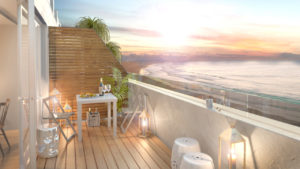## Margate_Balcony_Preview_002ProEXR File Description

=Attributes=
cameraAperture (float): 36.000000
cameraFarClip (float): 1000.000000
cameraFarRange (float): 1000.000000
cameraFov (float): 71.383331
cameraNearClip (float): 0.000000
cameraNearRange (float): 0.000000
cameraProjection (int): 0
cameraTargetDistance (float): 2000.000000
cameraTransform (m44f)
channels (chlist)
compression (compression): Zip
dataWindow (box2i): [0, 0, 1279, 719]
displayWindow (box2i): [0, 0, 1279, 719]
gamma (float): 1.000000
lineOrder (lineOrder): Increasing Y
pixelAspectRatio (float): 1.000000
screenWindowCenter (v2f): [0.000000, 0.000000]
screenWindowWidth (float): 1.000000

=Channels=
A (half)
AOCC.A (half)
AOCC.B (half)
AOCC.G (half)
AOCC.R (half)
B (half)
G (half)
R (half)
UVW.U (float)
UVW.V (float)
VRayGlobalIllumination.A (half)
VRayGlobalIllumination.B (half)
VRayGlobalIllumination.G (half)
VRayGlobalIllumination.R (half)
VRayLighting.A (half)
VRayLighting.B (half)
VRayLighting.G (half)
VRayLighting.R (half)
VRayNormals.A (half)
VRayNormals.B (half)
VRayNormals.G (half)
VRayNormals.R (half)
VRayRawReflection.A (half)
VRayRawReflection.B (half)
VRayRawReflection.G (half)
VRayRawReflection.R (half)
VRayReflection.A (half)
VRayReflection.B (half)
VRayReflection.G (half)
VRayReflection.R (half)
VRayRefraction.A (half)
VRayRefraction.B (half)
VRayRefraction.G (half)
VRayRefraction.R (half)
VRaySpecular.A (half)
VRaySpecular.B (half)
VRaySpecular.G (half)
VRaySpecular.R (half)
Z.B (float)
Z.G (float)
Z.R (float)